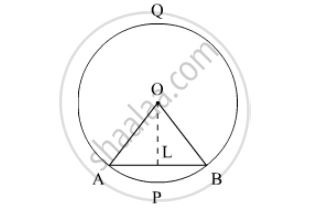Advertisement Remove all ads

# Ab is a Chord of a Circle with Centre O and Radius 4 Cm. Ab is of Length 4 Cm. Find the Area of the Sector of the Circle Formed by Chord Ab. - Mathematics

Sum

AB is a chord of a circle with centre O and radius 4 cm. AB is of length 4 cm. Find the area of the sector of the circle formed by chord AB.

Advertisement Remove all ads

#### Solution

We have to find the area of the sector AOB formed by the chord AB.We have  OA=4 cm and AB=4 cm. So,

AL=(AB)/2 cm

= 4/2 cm

= 2 cm

Let ∠AOB=2θ. then,

∠AOL=∠BOL

=θ

In ΔOLA, We have

sin θ=(AL)/(OA)

= 2/4

=1/2

θ = sin^-1  1/2

= 30°

Hence,  ∠AOB=60°

Now, using the value of∠AOB  and r we will find the area of sector AOB,

A=θ/(360°) xx pir^2

= (60°)/(360°)xx pixx4xx4 cm^2

=(8pi)/3 cm^2

Is there an error in this question or solution?
Advertisement Remove all ads

#### APPEARS IN

RD Sharma Class 10 Maths
Chapter 13 Areas Related to Circles
Exercise 13.2 | Q 24 | Page 25
Advertisement Remove all ads

#### Video TutorialsVIEW ALL 

Advertisement Remove all ads
Share
Notifications

View all notifications

Forgot password?
Course